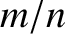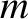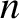# Introduction

As was explained in the previous two chapters, when describing the response of a tokamak plasma to a tearing mode perturbation, the plasma can be divided into two regions . In the so-called outer region, which comprises most of the plasma, the tearing perturbation is governed by the equations of marginally-stable ideal-MHD. However, these equations become singular at the so-called rational magnetic flux-surface where the safety-factor (see Section 3.2) takes the rational value. Here,andare the poloidal and toroidal mode numbers, respectively, of the tearing mode. (See Chapter 3.) A radially thin resistive layer forms around the rational magnetic flux-surface, and constitutes the so-called inner region. The aim of this chapter is to employ the reduced drift-MHD model derived in the previous chapter to determine the linear response of the plasma in the inner region to a rotating tearing perturbation in the outer region.